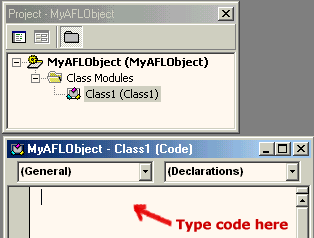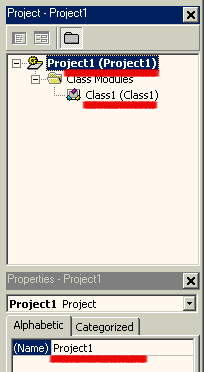## Component Object Model support in AFL

### Introduction

The Component Object Model (COM) is the technology that defines and implements mechanisms that enable software components, such as applications, data objects, controls, and services, to interact as objects. The COM support in AFL introduced in version 3.75beta allows to create instances of COM objects and call the functions (methods) exposed by those objects.

The COM object can be created in virtually any language including any C/C++ flavour, Visual Basic, Delphi, etc. This enables you to write parts of your indicators, systems, explorations and commentaries in the language of your choice and run them at full compiled code speed.

The scripting engines used by AFL scripting host (JScript and VBScript) also expose themselves as COM objects. AFL COM support now allows you to call functions defined in scripting part directly from AFL without the use of variables to pass the data to and retrieve data from the script.

### Calling functions defined in script

Until version 3.75 the only way to exchange information between AFL and the script was using variables - this technique is explained in detail in AFL scripting host documentation .

Let's suppose we need a function that calculates second order IIR (infinite impulse response) filter:

y[ n ] = f0 * x[ n ] + f1 * y[ n - 1 ] + f2 * y[ n - 2 ]

Please note that well known exponential smoothing is a first order IIR filter. Implementing higher order filters minimizes lag, therefore our second order IIR may be used as a "better" EMA.

In the "old way" we would need to write the following code:

`EnableScript("jscript");`

`x = ( High + Low )/2;`

```f0 = 0.2; f1 = 1.2; f2 = -0.4;```

```<% x = VBArray( AFL( "x" ) ).toArray(); f0 = AFL( "f0" ); f1 = AFL( "f1" ); f2 = AFL( "f2" );```

`y = new Array();`

```// initialize first 2 elements of result array y[ 0 ] = x[ 0 ]; y[ 1 ] = x[ 1 ]```

```for( i = 2; i < x.length; i++ ) {   y[ i ] = f0 * x[ i ] + f1 * y[ i - 1 ] + f2 * y[ i - 2 ]; }```

```AFL.Var("y") = y; %>```

```Graph0 = Close; Graph0Style = 64; Graph1 = y;```

While it is OK for one-time use, if we need such a function multiple times we had to have repeat the script part which is not very nice. Much nicer approach is to have a function that can be called from multiple places without the need to repeat the same code. Defining functions in JScript of VBScript is no problem at all:

```EnableScript("jscript"); ```

```<% ```

```function IIR2( x, f0, f1, f2 ) {```

`x = VBArray( x ).toArray();`

`y = new Array();`

```// initialize first 2 elements of result array y[ 0 ] = x[ 0 ]; y[ 1 ] = x[ 1 ];```

```for( i = 2; i < x.length; i++ ) {    y[ i ] = f0 * x[ i ] + f1 * y[ i - 1 ] + f2 * y[ i - 2 ]; }```

`return y;`

`} `

`%>`

.. but how to call such a function from AFL?

The most important thing is that script engine exposes itself as a COM object. A new AFL function GetScriptObject() can be used to obtain the access to the script engine. The rest is simple - once we define the function in the script it is exposed as a method of script object retrieved by GetScriptObject:

```script = GetScriptObject(); Graph0 = script.IIR2( ( High + Low )/2, 0.2, 1.2, -0.4 ); Graph1 = script.IIR2( ( Open + Close )/2, 0.2, 1.0, -0.2 ); // call it again and again...```

Note also, that with this approach we may pass additional arguments so our IIR2 filter may be re-used with various smoothing parameters.

So, thanks to a new COM support in AFL, you can define functions in scripts and call those functions from multiple places in your formula with ease.

### Using external COM/ActiveX objects in AFL

In a very similar way we can call functions (methods) in an external COM objects directly from the AFL formula. Here I will show how to write such external ActiveX in Visual Basic but you can use any other language for this (Delphi for example is very good choice for creating ActiveX/COM objects).

It is quite easy to create your own ActiveX DLL in Visual Basic, here are the steps required:

 Run Visual Basic In the "New project" dialog choose "ActiveX DLL" icon - this will create the "Project1" that looks like in the picture on the right: Now click on the (Name) and rename the "Project1" to something more meaningfull, for example "MyAFLObject" Then double click on the "Class1" in the project tree item. The code window will get the title of "MyAFLObject - Class1 (Code)" as shown below:Now you are ready to enter the codeAs an example we will implement a similar function to one shown in the JScript. The function will calculate second order Infinite Impulse Response filter. We will call this function "IIR2"

```Public Function IIR2(InputArray() As Variant, f0 As Variant, f1 As Variant, f2 As Variant) As Variant```

`Dim Result()`

`ReDim Result(UBound(InputArray)) ' size the Result array to match InputArray`

`'initialize first two elements`

```Result(0) = InputArray(0) Result(1) = InputArray(1)```

`For i = 2 To UBound(InputArray)`

```   Result(i) = f0 * InputArray(i) + f1 * Result(i - 1) + f2 * Result(i - 2)```

`Next`

`IIR2 = Result`

`End Function`

The code is quite similar to the JScript version. The main difference is declaring types. As you can see all variables passed from and to AFL must be declared as Variants. This is so, because AmiBroker does not know what kind of object it speaks to and puts all arguments to the most universal Variant type and expects the function to return the value as Variant also. Currently AmiBroker can pass to your object floating point numbers, arrays of floating point numbers, strings, and pointers to other objects (dispatch pointers) - all of them packed into Variant type. When you write the ActiveX, it is your responsibility to interpret Variants received from AmiBroker correctly.

Now you should choose Run->Start in Visual Basic to compile and run the component. The code in Visual Basic will wait until external process accesses the code.

To access the freshly created ActiveX we will use the following AFL formula (enter it in the Formula Editor and press Apply):

`myobj = CreateObject("MyAFLObject.Class1");`

```Graph0 = Close; Graph0Style = 64; Graph1 = myobj.IIR2( Close, 0.2, 1.2, -0.4 );```

The AFL formula simply creates the instance of our ActiveX object and calls its member function (IIR2). Note that we are using new dot (.) operator to access myobj members.
Now click the "Apply" button in the Formula Editor to see how all this setup works. You should see candlestick chart with a quite nice moving average.

2.4 Conclusion

Introduction of COM support in AFL brings even more power to AFL and AmiBroker. Now you can write indicators, trading systems, explorations and commentaries using custom functions that are easy to create using scripting language or full-featured development environment of Visual Basic, Borland Delphi, C++ Builder, Visual C++ and many, many others. Using integrated development environments like those mentioned makes debugging, testing and developing much easier and faster. Also resulting compiled code executes several times faster than interpreted script or AFL.

But this is not the end of the story... C/C++ programmers can choose to write plugin DLLs that do not use COM technology at all. Plugin DLLs has some additional features including ability to call back AFL built-in functions, directly retreive and set AFL variables and support automatic syntax colouring of functions exposed by the plugin. This topic is covered in the AmiBroker Development Kit available from the member's area of AmiBroker site.